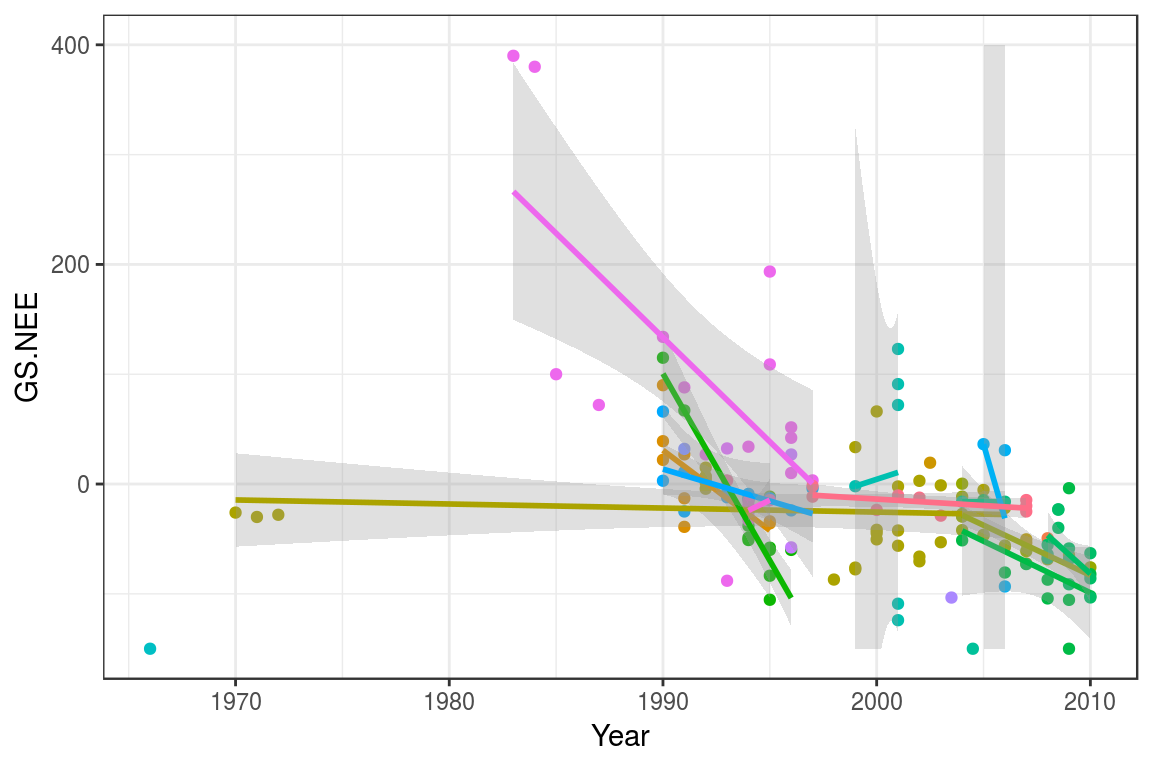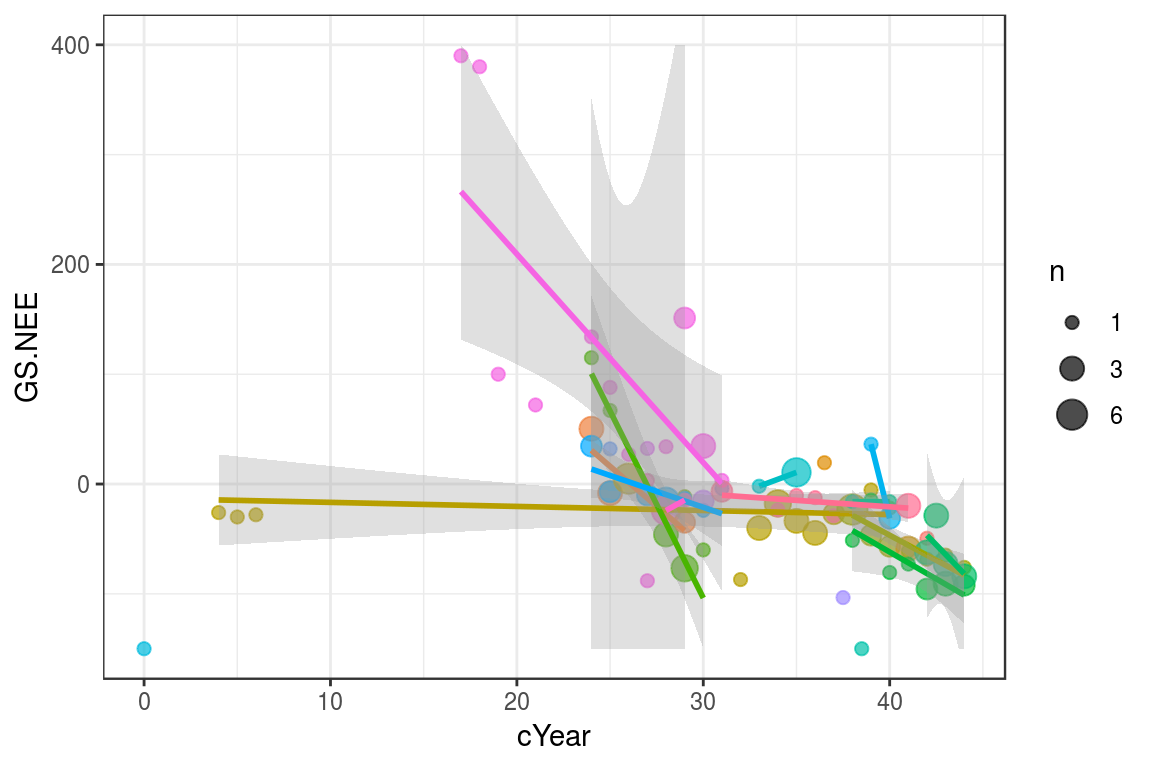# Introduction

These are worked examples for a book chapter on mixed models in Ecological Statistics: Contemporary Theory and Application editors Negrete, Sosa, and Fox (available from the Oxford University Press catalog or from Amazon.com or Powell’s Books or …).

Data and source code for this file are currently available at Github.

There’s a lot of material here. I have erred on the side of including things, and on the side of compact rather than elementary code. Try not to be overwhelmed, just skim it the first time and thereafter focus on the parts that are most relevant to your analyses.

I use a very large set of packages in this example, because I am trying out a wide range of approaches. You should whittle these down to just the ones you need for any given analysis … if you want to install all of them at once, the following code should be sufficient (remember that you only have to install packages one time on each computer you are using, but you have to use library() to load them in every new R session). The packages noted below as “not on CRAN” (glmmADMB) are hosted on R-forge (http://r-forge.r-project.org), but in order to install them you may have to install from http://www.math.mcmaster.ca/bolker/R, as below.

pkgs_CRAN <- c("lme4","MCMCglmm","blme",
"pbkrtest","coda","aods3","bbmle","ggplot2",
"reshape2","plyr","numDeriv","Hmisc",
"broom.mixed","dotwhisker")
install.packages(pkgs_CRAN)
rr <- "http://www.math.mcmaster.ca/bolker/R"
library("devtools")
## primary GLMM-fitting packages:
library("lme4")
library("glmmTMB")
library("MCMCglmm")
library("blme")
library("MASS")          ## for glmmPQL (base R)
library("nlme")          ## for intervals(), tundra example (base R)
## auxiliary
library("ggplot2")       ## for pretty plots generally
## ggplot customization:
theme_set(theme_bw())
scale_colour_discrete <- function(...,palette="Set1") {
scale_colour_brewer(...,palette=palette)
}
scale_colour_orig <- ggplot2::scale_colour_discrete
scale_fill_discrete <- function(...,palette="Set1") {
scale_fill_brewer(...,palette=palette)
}
## to squash facets together ...
zmargin <- theme(panel.spacing=grid::unit(0,"lines"))
library("gridExtra")     ## for grid.arrange()
library("broom.mixed")
## n.b. as of 25 Sep 2018, need bbolker github version of dotwhisker ...
library("dotwhisker")
library("coda")      ## MCMC diagnostics
library("aods3")     ## overdispersion diagnostics
library("plotMCMC") ## pretty plots from MCMC fits
library("bbmle")     ## AICtab
library("pbkrtest")  ## parametric bootstrap
library("Hmisc")
## for general-purpose reshaping and data manipulation:
library("reshape2")
library("plyr")
## for illustrating effects of observation-level variance in binary data:
library("numDeriv")

Package versions used (core packages only):

##       lme4   glmmADMB   MCMCglmm       blme   pbkrtest       coda
##   1.1.18.1      0.8.5       2.26      1.0.4      0.4.7     0.19.1
##      aods3      bbmle    glmmTMB
##    0.4.1.1     1.0.21 0.2.2.9000

If you are using Windows and have problems using glmmADMB on some problems, there is a (slightly tedious) workaround:

• check the directory that contains the latest version of the glmmADMB binary component; find the most recent version of the binaries for your operating system (Windows, MacOS, Linux [Fedora or Ubuntu], and 32 vs 64 bits: check sessionInfo()$platform for more information). For example, the most recent Windows binary as of this writing is glmmadmb-mingw64-r2885-windows8-mingw64.exe. If you find more than one file that seems to apply, just pick one at random. • Once you’ve figured out what file to download, execute the following code (substituting the name of the appropriate binary file in the last line): library("glmmADMB") bb <- glmmADMB:::get_bin_loc()[["bin_loc"]] bpath <- gsub("glmmadmb$","",bb)
"glmmadmb-mingw64-r2885-windows8-mingw64.exe"), dest=bb)

# Tundra carbon

These data were originally analyzed in Belshe et al. (2013).

## Data exploration

mc1 <- read.csv("data/tundra.csv",na.strings=c("-","NA"))
V1 V2
Year year of data collection
Site location
Lat latitude
Long longitude
Method ?
GS.NEE net ecosystem exchange, growing season
GS.GPP gross primary production, growing season
GS.ER ecosystem respiration, growing season
W.NEE net ecosystem exchange, winter
MAT ?
TAP ?
Reference Literature reference

A first look at the data, plotting net ecosystem exchange during the growing season (GS.NEE) against year, using colour to distinguish sites, and superimposing a separate linear regresssion (with confidence bands) per site:

ggplot(mc1,aes(x=Year,y=GS.NEE,colour=Site))+geom_point()+
geom_smooth(method="lm",alpha=0.3)+
## oob=squish retains the (truncated) confidence bands;
## otherwise bands that went outside the limits would disappear
scale_y_continuous(limits=c(-150,400),oob=scales::squish)+
## use original colours (Dark2 doesn't have enough distinct values)
## suppress legend
scale_colour_orig(guide="none")We have suppressed the legend for the colours, because there are a lot of sites and their names will not be particularly meaningful except to tundra biologists. There is lots of variability among sites, both in the trend and in the uncertainty of the trend. The uncertainty is caused in part by noisiness of the data, and part by sparsity/shortness of the time series for individual sites.

In some cases there were multiple observations per site in a single year. We could have handled this by adding a year-within-site random variable, but it proved to be easier to aggregate these cases by calculating the mean, and account for the different amount of sampling by weighting site-year combinations according to the number of samples.

The following (rather complicated) function aggregates the data; in the course of our analysis we ended up needing to aggregate several different response variables according to several different sets of grouping variables. We re-centred the year to have year=0 at the beginning of the observation period.

aggfun <- function(dat,agg=c("Year","Site"),response="Winter.adj",
baseYear=min(mc1$Year)) { ## select only site, year, and response sub1 <- na.omit(dat[,c("Site","Year",response)]) ## compute means of non-aggregation variables agg1 <- aggregate(sub1[!names(sub1) %in% agg],by=sub1[agg],FUN=mean) ## compute sample size of non-aggregation variables aggn <- aggregate(sub1[response],by=sub1[agg],FUN=length) names(aggn)[ncol(aggn)] <- "n" ## assumes response is last column ## put mean and sample size together agg2 <- merge(agg1,aggn) ## recompute centred year agg2$cYear <- agg2$Year - baseYear agg2 } mc2 <- aggfun(mc1,response="GS.NEE") ## center year at the mean rather than the date of ## the first observation: mc2B <- aggfun(mc1,response="GS.NEE",baseYear=mean(mc1$Year))

The aggregated data show a similar picture:

ggplot(mc2,aes(x=cYear,y=GS.NEE,colour=Site))+
geom_point(aes(size=n),alpha=0.7)+
geom_smooth(method="lm",alpha=0.3,aes(weight=n))+
scale_y_continuous(limits=c(-150,400),oob=scales::squish)+
scale_colour_orig(guide="none")+
scale_size_continuous(range=c(2,5),breaks=c(1,3,6))## Fitting

We use nlme::lme because at present it is the only easy way to allow for temporal autocorrelation in a LMM in R.

• we use corCAR1, which implements a continuous-time first-order autocorrelation model (i.e. autocorrelation declines exponentially with time), because we have missing values in the data. The more standard discrete-time autocorrelation models (lme offers corAR1 for a first-order model and corARMA for a more general model) don’t work with missing data.
• The weights=varFixed(~I(1/n)) specifies that the residual variance for each (aggregated) data point is inversely proportional to the number of samples.
• The control argument lets the model try more iterations (otherwise we get an error).
cmod_lme <- lme(GS.NEE ~ cYear,
data=mc2, method="REML",
random = ~ 1 + cYear | Site,
correlation=corCAR1(form=~cYear|Site),
weights=varFixed(~I(1/n)),
control=list(maxIter=10000, niterEM=10000))

Model summary:

summary(cmod_lme)
## Linear mixed-effects model fit by REML
##  Data: mc2
##       AIC      BIC   logLik
##   879.754 896.4282 -432.877
##
## Random effects:
##  Formula: ~1 + cYear | Site
##  Structure: General positive-definite, Log-Cholesky parametrization
##             StdDev     Corr
## (Intercept) 147.904084 (Intr)
## cYear         4.231723 -1
## Residual     60.062783
##
## Correlation Structure: Continuous AR(1)
##  Formula: ~cYear | Site
##  Parameter estimate(s):
##       Phi
## 0.4039311
## Variance function:
##  Structure: fixed weights
##  Formula: ~I(1/n)
## Fixed effects: GS.NEE ~ cYear
##                 Value Std.Error DF   t-value p-value
## (Intercept) 108.88882  48.02267 57  2.267446  0.0272
## cYear        -3.85221   1.37468 57 -2.802256  0.0069
##  Correlation:
##       (Intr)
## cYear -0.987
##
## Standardized Within-Group Residuals:
##        Min         Q1        Med         Q3        Max
## -2.7595981 -0.4217636 -0.1201307  0.2685378  3.0854580
##
## Number of Observations: 82
## Number of Groups: 24

The results generally look sensible: the only warning sign is that the among-site variation in baseline NEE ((Intercept)) and the among-site variation in slope are perfectly correlated (i.e., the -1 term in the Corr column under Random effects). We weren’t happy with this, but we kept the full random effects model anyway. The alternative, dropping the random effect of year, seemed unacceptable in our case. Recentring the data at the mean year ($$\approx$$ 1997) improves things slightly:

cmod2_lme <- update(cmod_lme,data=mc2B)

VarCorr() or summary() show that the intercept and slope random effects are still very strongly, but not perfectly, correlated ($$\rho=0.973$$); the fixed-effect intercept is very different (of course), and the year effect is almost identical, but its standard error is larger (so its $$p$$-value doubles).

##              Value Std.Error DF t-value p-value
## (Intercept) -17.42      7.77 57   -2.24   0.029 *
## cYear        -3.84      1.51 57   -2.55   0.014 *
## ---
## Signif. codes:  0 '***' 0.001 '**' 0.01 '*' 0.05 '.' 0.1 ' ' 1

We can also fit the model with lmer from the lme4 package: it’s faster and allows for crossed random effects (neither of which really matters here), but unfortunately it can’t incorporate temporal autocorrelation in the model:

cmod_lmer <- lmer(GS.NEE ~ cYear + (1+cYear|Site),
data=mc2B, REML=TRUE,
weights=n)
summary(cmod_lmer)
## Linear mixed model fit by REML ['lmerMod']
## Formula: GS.NEE ~ cYear + (1 + cYear | Site)
##    Data: mc2B
## Weights: n
##
## REML criterion at convergence: 874.2
##
## Scaled residuals:
##      Min       1Q   Median       3Q      Max
## -2.90210 -0.35039 -0.07972  0.30152  2.93146
##
## Random effects:
##  Groups   Name        Variance Std.Dev. Corr
##  Site     (Intercept)  116.03  10.771
##           cYear         19.95   4.466   -1.00
##  Residual             3355.31  57.925
## Number of obs: 82, groups:  Site, 24
##
## Fixed effects:
##             Estimate Std. Error t value
## (Intercept)  -16.296      7.338  -2.221
## cYear         -3.745      1.341  -2.792
##
## Correlation of Fixed Effects:
##       (Intr)
## cYear -0.417

Note that weights here are specified as n rather than 1/n (varFixed() in the lme call specifies the variance, rather than the actual weights of different observations)

The results are not too different – the cYear fixed-effect slope is slightly smaller than for the lme fit (-3.75 vs. -3.84 (g C m^2 /year/season)/year), but the standard error is smaller, so the $$t$$-statistic is similar (-2.79 vs. -2.55).

cmod_glmmTMB <- glmmTMB(GS.NEE ~ cYear + (1+cYear|Site),
data=mc2B,
weights=n)
## Warning in fitTMB(TMBStruc): Model convergence problem; non-positive-
## definite Hessian matrix. See vignette('troubleshooting')
summary(cmod_glmmTMB)
##  Family: gaussian  ( identity )
## Formula:          GS.NEE ~ cYear + (1 + cYear | Site)
## Data: mc2B
## Weights: n
##
##      AIC      BIC   logLik deviance df.resid
##       NA       NA       NA       NA       76
##
## Random effects:
##
## Conditional model:
##  Groups   Name        Variance  Std.Dev. Corr
##  Site     (Intercept) 3.841e-02  0.196
##           cYear       3.148e+01  5.611   -0.79
##  Residual             1.543e+03 39.280
## Number of obs: 82, groups:  Site, 24
##
## Dispersion estimate for gaussian family (sigma^2): 1.54e+03
##
## Conditional model:
##             Estimate Std. Error z value Pr(>|z|)
## (Intercept)  -22.347      5.579  -4.005 6.19e-05 ***
## cYear         -3.706      1.416  -2.617  0.00887 **
## ---
## Signif. codes:  0 '***' 0.001 '**' 0.01 '*' 0.05 '.' 0.1 ' ' 1

Compare parameters (not yet working!)

dwplot(list(glmmTMB=cmod_glmmTMB,lmer=cmod_lmer),by_2sd=TRUE)

We have to admit that the model we are using is just a little bit more complex than the data can easily handle, and especially that Toolik is quite different from the other sites … making the estimates somewhat unstable.

## Diagnostics

Here are the default residuals-vs.-fitted plot; the scale-location plot ($$\sqrt{|\textrm{residual}|}$$ vs. fitted); a plot of the residuals as a function of year; and the Q-Q plot.

colvec <- c("#ff1111","#007eff") ## second colour matches lattice default
grid.arrange(plot(cmod2_lme,type=c("p","smooth")),
plot(cmod2_lme,sqrt(abs(resid(.)))~fitted(.),
col=ifelse(mc2$Site=="Toolik, AK",colvec,colvec), type=c("p","smooth"),ylab=expression(sqrt(abs(resid)))), ## "sqrt(abs(resid(x)))"), plot(cmod2_lme,resid(.,type="pearson")~cYear, type=c("p","smooth")), qqnorm(cmod2_lme,abline=c(0,1), col=ifelse(mc2$Site=="Toolik, AK",colvec,colvec)))